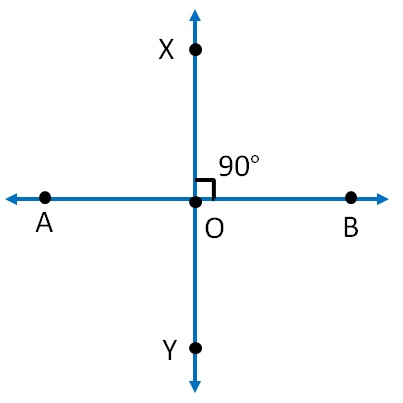Perpendicular Lines

Chapter 5 Class 6 Understanding Elementary Shapes
Concept wise

Let’s take lines AB & XYNow,

XY ⊥ AB

If XY bisects AB as well

i.e. if O is the mid-point of AB

i.e. if OA = OB

Then, XY is perpendicular bisector of AB.

Perpendicular bisector means

• Line is perpendicular
• Line bisects the other line

Here,

• XY ⊥ AB
• And OA = OB

∴  XY is perpendicular bisector of AB.

Learn in your speed, with individual attention - Teachoo Maths 1-on-1 Class